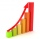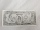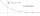# Savings

Suppose on your 21st birthday you begin making monthly payments of $500 into an account that pays 8% compounded monthly. If you continue the payments untill your 51st birthday (30 years), How much money will be in your account? How much of it is interest? Show solution. (Future value with regular contributions, future value of a series) ### Correct answer: x = 745179.7243 i = 565179.7243 ### Step-by-step explanation:Did you find an error or inaccuracy? Feel free to write us. Thank you!Tips to related online calculators Do you want to convert time units like minutes to seconds? #### You need to know the following knowledge to solve this word math problem: ## Related math problems and questions: • Future valueSuppose you invested$1000 per quarter over a 15 years period. If money earns an anual rate of 6.5% compounded quarterly, how much would be available at the end of the time period? How much is the interest earn?
• If you 2If you deposit $4000 into an account paying 9% annual interest compounded monthly, how long until there is$10000 in the account?
• Present valueA bank loans a family $90,000 at 4.5% annual interest rate to purchase a house. The family agrees to pay the loan off by making monthly payments over a 15 year period. How much should the monthly payment be in order to pay off the debt in 15 years? • How much 2How much money would you need to deposit today at 5% annual interest compounded monthly to have$2000 in the account after 9 years?
• If you 4If you deposit $2500 in an account paying 11% annual interest compounded quarterly, how long until there is the$4500 in the account?
• Five yearsNakato Nobuki, a Japanese artist, wants to have $24,000.00 in his savings account at the end of five years. Mr. Nobuki deposits$1,500.00 annually into savings and has a balance of $8,000.00 today. What must the interest rate on the savings account be for • Suppose 3Suppose that a couple invested Php 50 000 in an account when their child was born, to prepare for the child's college education. If the average interest rate is 4.4% compounded annually, a, Give an exponential model for the situation b, Will the money be • Semiannually compound interestIf you deposit$5000 into an account paying 8.25% annual interest compounded semiannually, how long until there is $9350 in the account? • If you 3If you deposit$4500 at 5% annual interest compound quarterly, how much money will be in the account after 10 years?
• Future value investmentPoseidon deposited 2,747 golden drachmas in a Mount Olympus college savings account to ensure Percy can go to college. It pays 0.04 (percent in decimal form) annual interest. After 11 years, he withdraws the money. How much more money would he have if the
• Compound InterestIf you deposit $6000 in an account paying 6.5% annual interest compounded quarterly, how long until there is$12600 in the account?
• Saving 9An amount of $2000 is invested at an interest of 5% per month. If$ 200 is added at the beginning of each successive month but no withdrawals. Give an expression for the value accumulated after n months. After how many months will the amount has accumula
• Compound interest 43600 dollars is placed in an account with an annual interest rate of 9%. How much will be in the account after 25 years, to the nearest cent?
• InterestCalculate how much you earn for $n years$x deposit if the interest rate is $p% and the interest period is a quarter. • Retirement annuityHow much will it cost to purchase a two-level retirement annuity that will pay$2000 at the end of every month for the first 10 years, and \$3000 per month for the next 15 years? Assume that the payment represent a rate of return to the person receiving th
• You takeYou take out Php 20 000 loan at 5% interest rate. If the interest is compounded annually, a. Give an exponential model for the situation b. How much Will you owe after 10 years?
• Suppose 2Suppose that the half-life of a substance is 250 years. If there were initially 100 g of the substance, a. Give an exponential model for the situation b. How much will remain after 500 years?